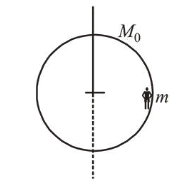# A person of 80 kg mass is standing on the rimQuestion:

A person of $80 \mathrm{~kg}$ mass is standing on the rim of a circular platform of mass $200 \mathrm{~kg}$ rotating about its axis at 5 revolutions per minute $(\mathrm{rpm})$. The person now starts moving towards the centre of the platform. What will be the rotational speed (in rpm) of the platform when the person reaches its centre___________

Solution:

$(9.00)$

Here $M_{0}=200 \mathrm{~kg}, m=80 \mathrm{~kg}$

Using conservation of angular momentum, $L_{i}=L_{f}$$I_{1} \omega_{1}=I_{2} \omega_{2}$

$I_{1}=\left(I_{M}+I_{m}\right)=\left(\frac{M_{0} R^{2}}{2}+m R^{2}\right)$

$I_{2}=\frac{1}{2} M_{0} R^{2}$ and $\omega_{1}=5 \mathrm{rpm}$

$\therefore \omega_{2}=\left(\frac{M_{0} R^{2}}{2}+m R^{2}\right) \times \frac{5}{\frac{M_{0} R^{2}}{2}}$

$=\frac{5 R^{2}}{R^{2}} \times \frac{(80+100)}{100}=9 \mathrm{rpm}$